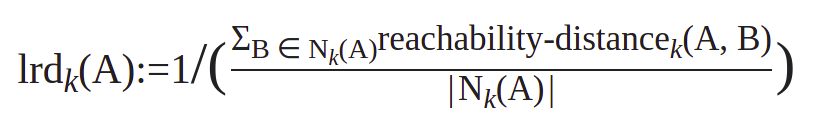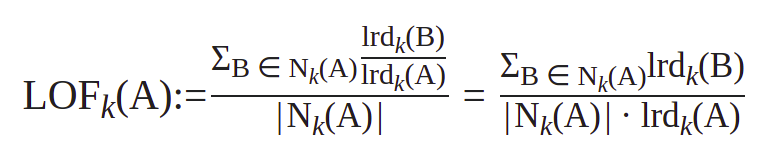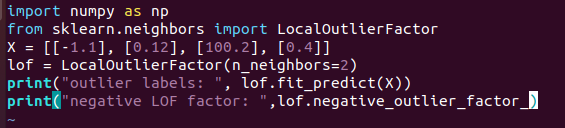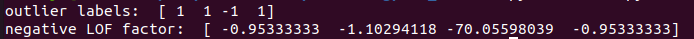# Local Outlier Factor for Anomaly Detection

Anomaly detection is an important application used across various verticals like healthcare, finance, manufacturing and so on. Local Outlier Factor is a popular density based technique for anomaly detection that does not require prior examples of anomalies.

## What are Advantages of LOF for Anomaly Detection?

• One of the main challenges with Anomaly detection is the lack of training data specifying examples of anomalies to train models for predicting them. LOF is an unsupervised technique that does not require prior examples.
• Several of the simple distance based models for anomaly detection fail when the data has different density in different regions. i.e there are areas where points are close together and areas where points are spread out in the dataset. The LOF method can handle such situations
• Many of the anomaly detection algorithms fail for multimodal distrubutions. LOF can work well for such data as well.

## How does Local Outlier Factor Method Work?

The local outlier factor method works by comparing the density of a point with the relative densities of its neighbours. If a point is relatively less dense than its neighbours, it is a potential outlier. If the ratio of density of neighbours to the density of point is too high, we end up with a high LOF signifying an outlier.

Now How to compute the density of a point?

We first define the K-distance of a point. This is intuitively the distance of the Kth nearest neighbour to the point.

K-distance(A)= Dist(A, Kth nearest neighbour)

The K neighbourhood of a point is just the K closest points to a given point.

Nk(A) = { P | Dist(A,P)<= K-distance(A)}

The reachability distance defines how easy it is to reach a point from another point. If a point A is in the K-neighbourhood of B then it is easier to reach A and the reachability distance is lower. For a point A that is not in the K-neighbourhood of B, the reachability distance is higher.

Reachability Distancek(A,B)= max{ k-distance(B), dist(A,B) }

The local reachability density is defined as the reciprocal of the average reachability distance of points around A to A:Now that we know the density of a point, the local reachability factor is just the average ratio of densities of neighbouring points of A to A.Note that by taking the ratio of density with neighbouring points and not the actual densities themselves, the LOF method can handle datasets with regions of different densities.

## Python Code for Local Outlier Factor Method

Here is a small toy example to show how LOF can be incorporated in your code. The array X has four points where one of the points 100.2 is a clear outlier. The LOF method can be called to identify outliers.We see the output of outlier labels that clearly shows the third data point as an outlier while the other points are not. The next line shows the negative of LOF value and we again see that the third point has a significantly lower negative value while others have something close to -1. Points that significantly deviate from 1 are outliers.